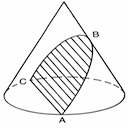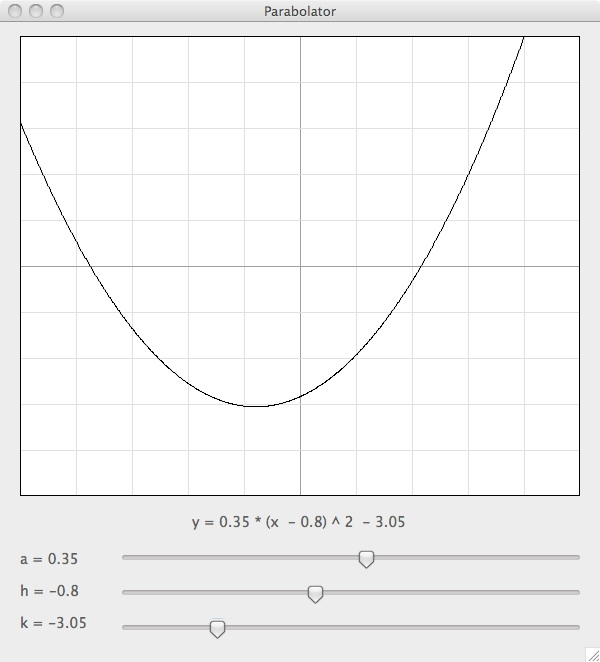## Parabolator

Demonstrates the parabola formula in vertex form.

Other Kaleberg SoftwareThis is a very simple program that does one thing and one thing only. It demonstrates how the parabola formula works in vertex form. That's the y = a * (x - h) ^ 2 + k form, where h is the x coordinate of the vertex, k is the y coordinate of the vertex and a is the scale factor for the parabola. As you can see in the screenshot below, it's a pretty simple program with a parabola and three sliders:There is a downloadable version for MacOS (Universal) and one for Windows:

This is another fine software product from Kaleberg Labs.

We have other useful software including a tide calculator for iCal, a tide finder, a volvelle maker, an equation solver and a slow motion Cardiac computer emulator.